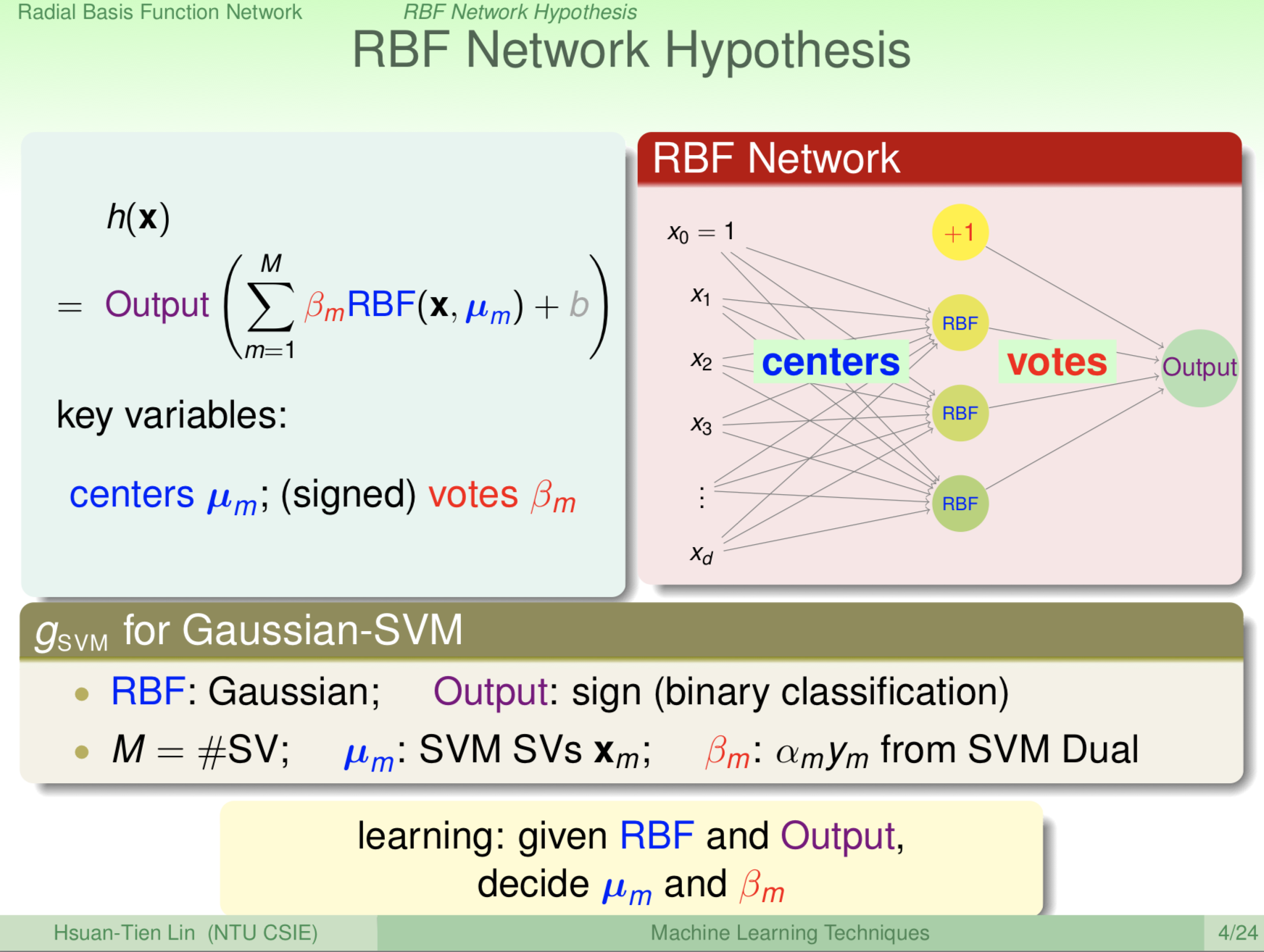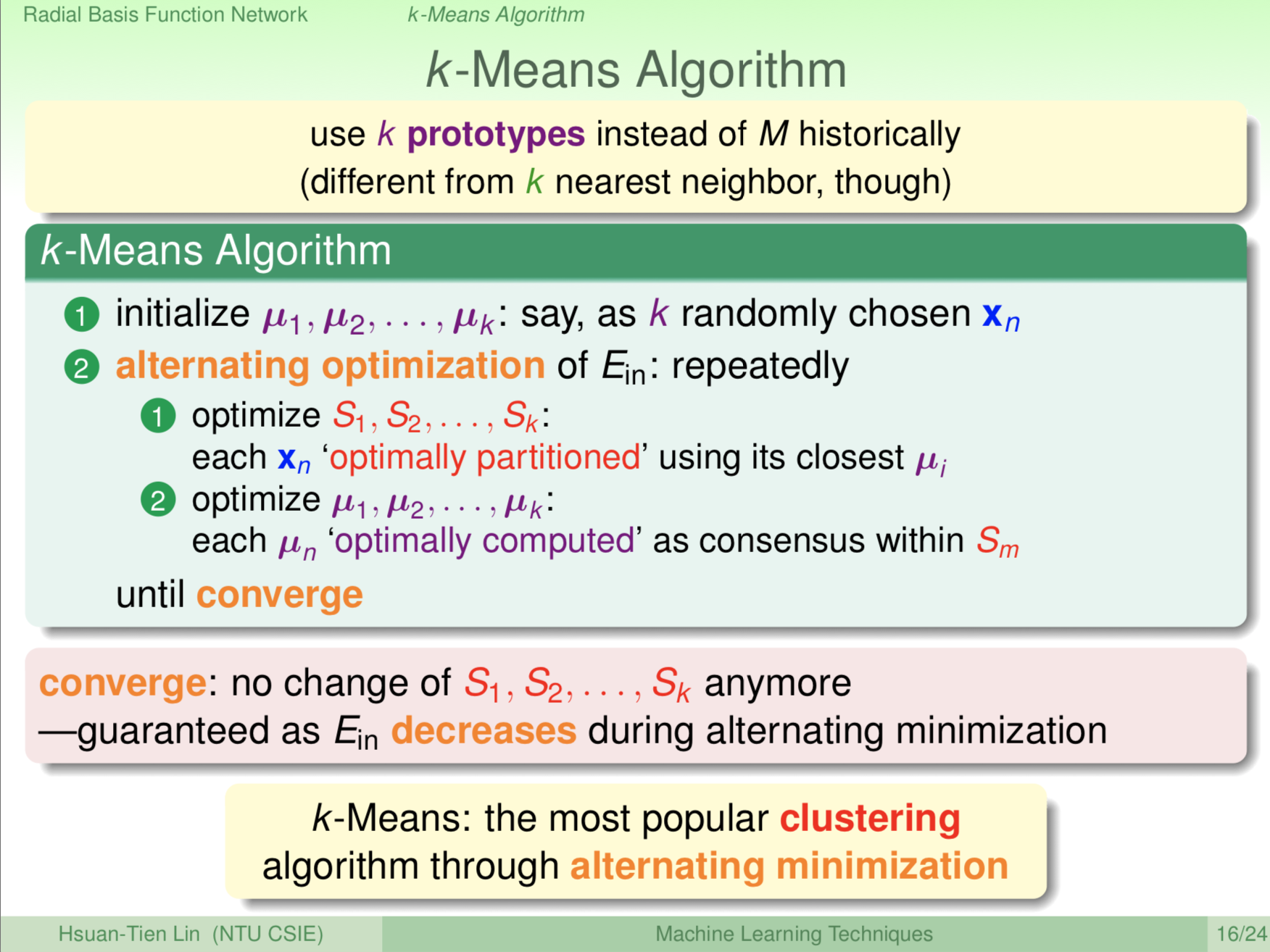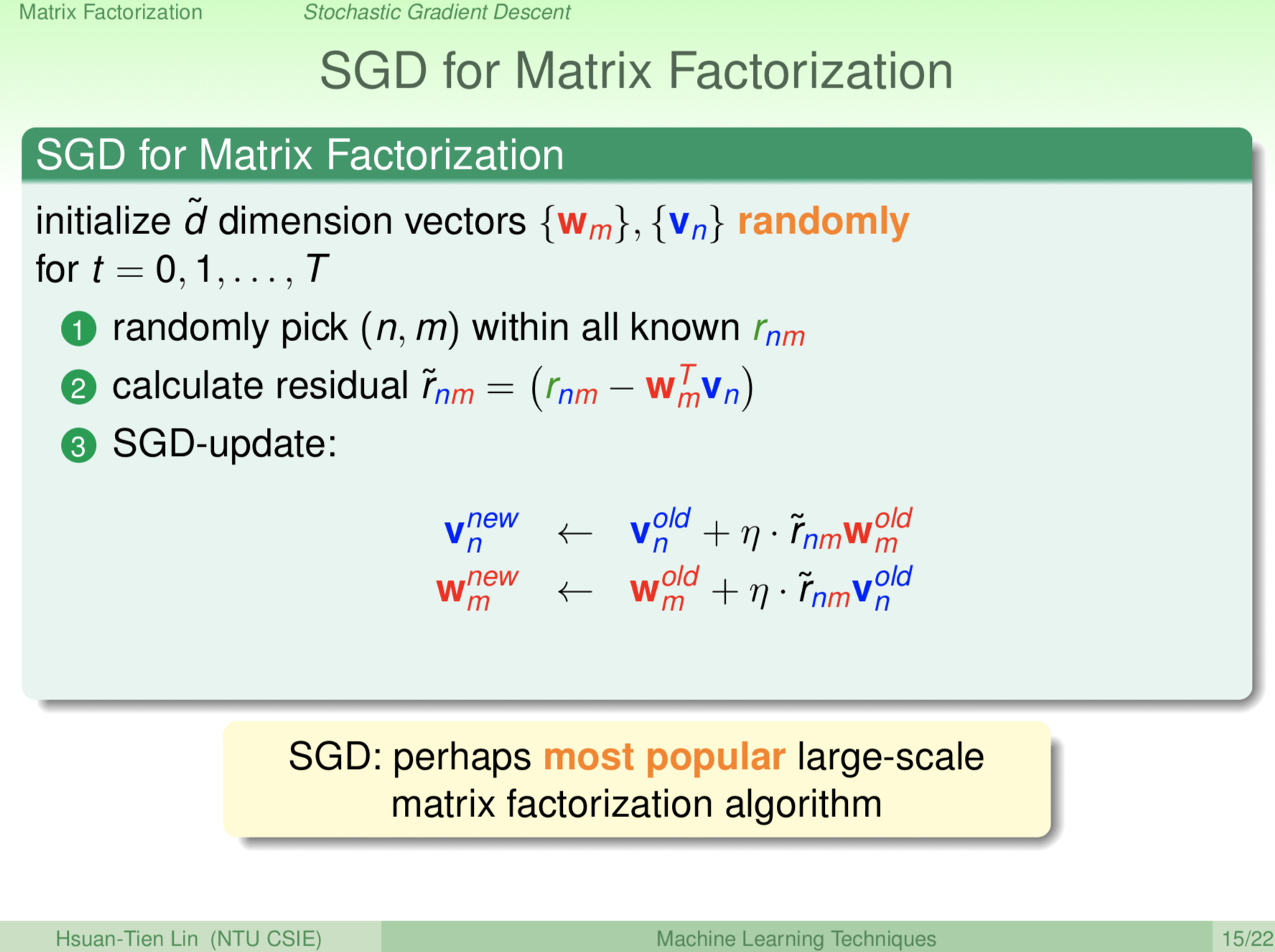# 機器學習技法 學習筆記 (7)：Radial Basis Function Network與Matrix Factorization

### Radial Basis Function (RBF) Network

$$W = 𝚺_{n=sv} α_n y_n Z_n$$

$$G_{SVM}$$

$$= sign[WZ+b]$$

$$= sign{[𝚺_{n=sv} α_n y_n K(X_n,X)]+b}$$

$$⇒ G_{SVM} = sign{[𝚺_{n=sv} α_n y_n exp(-γ |X-X_n|^2)]+b}$$

RBF Function: $$RBF(X,X_n)=exp(-γ|X-X_n|^2)$$

$$G=Output{[𝚺_m β_m RBF(X,μ_m)]+b}$$

$$Output$$$$sign$$ Function、$$β_m$$$$α_n y_n$$就回到特例SVM了。RBF Network在歷史上是Neural Network的一個分支，不過從上面的介紹你就會發現，它們的結構是有差異的，演算法也就不一樣。

### K-Means### One-Hot Encoding

$$A = [1, 0, 0, 0]^T$$

$$B = [0, 1, 0, 0]^T$$

$$AB = [0, 0, 1, 0]^T$$

$$O = [0, 0, 0, 1]^T$$

### Matrix Factorization

Matrix Factorization精神上有點像是Autoencoder，Autoencoder找出隱含在Data裡的特性，而Matrix Factorization則是找出隱含的匹配關係。Hypothesis: $$h(X) = W^TVX$$

$$h(X_n) = W^TV_n$$

$$W_m^T V_n ≈r_{nm}$$

$$E_{in}(\{W_m\},\{V_n\}) = (1/𝚺_m |D_m|)×𝚺_{n,m} (r_{nm}-W_{m}^TV_n)^2$$

### Alternating Least Squares for Matrix Factorization### SGD for Matrix Factorization$$E_{in}(\{W_m\},\{V_n\}) = (1/𝚺_m |D_m|) \times 𝚺_{n,m} (r_{nm}-W_{m}^T V_n )^2$$

### 結語

Matrix Factorization和Autoencoder有點類似，Autoencoder目標在於找出隱含在Data裡的特性，而Matrix Factorization則是找出隱含的匹配關係，並且介紹了兩種Matrix Factorization的演算法：Alternating Least Squares和SGD方法。

https://www.csie.ntu.edu.tw/~htlin/course/mltech17spring/doc/216_handout.pdf